——刘慈欣 《诗云》

doc = read.csv("SongPoem.csv");
author = doc$Author; cipai = doc$Title2;
tab = table(author, cipai);

r1 = sort(table(author), decreasing = TRUE)[1:20];
r1 = data.frame(Author = names(r1), Freq = r1);
rownames(r1) = 1:nrow(r1);
r1

r2 = sort(table(cipai), decreasing = TRUE)[1:20];
r2 = data.frame(Cipai = names(r2), Freq = r2);
rownames(r2) = 1:nrow(r2);
r2


Author Freq
1  <无名氏> 1569
2   辛弃疾  629
3     苏轼  362
4   刘辰翁  356
5   吴文英  341
6   赵长卿  339
7     张炎  302
8     贺铸  283
9   刘克庄  269
10  晏几道  260
11    吴潜  258
12  朱敦儒  246
13  欧阳修  242
14  张孝祥  224
15    柳永  213
16  陈允平  209
17    毛滂  204
18  李曾伯  202
19    韩淲  197
20  黄庭坚  192


Cipai Freq
1      浣溪沙  814
2    水调歌头  711
3      鹧鸪天  641
4      菩萨蛮  603
5      念奴娇  590
6      满江红  529
7      西江月  492
8      临江仙  477
9      蝶恋花  476
10 减字木兰花  441
11     贺新郎  438
12     沁园春  432
13     点绛唇  388
14   <失调名>  371
15     清平乐  346
16     满庭芳  325
17     玉楼春  308
18     水龙吟  305
19     虞美人  298
20     好事近  296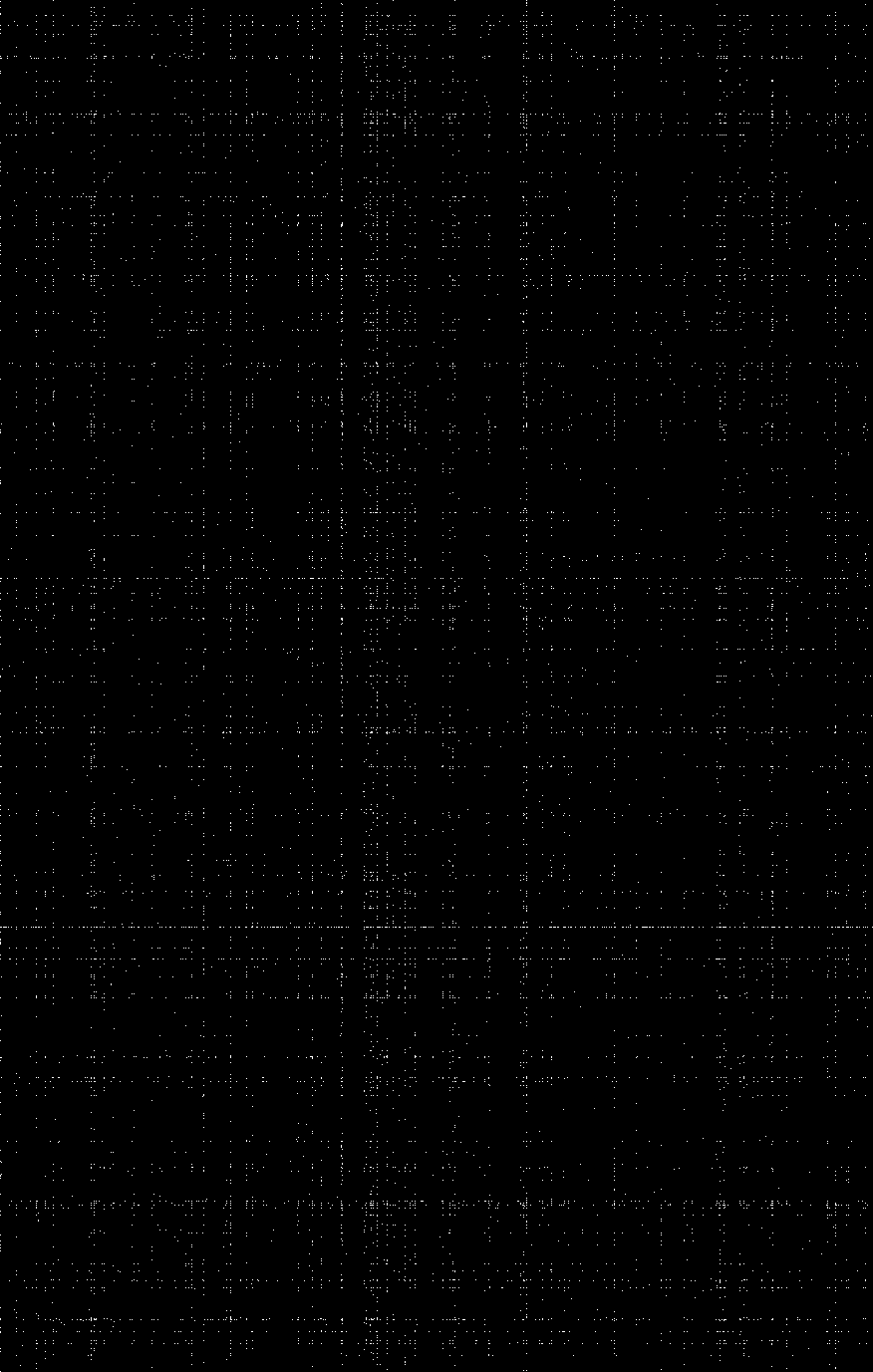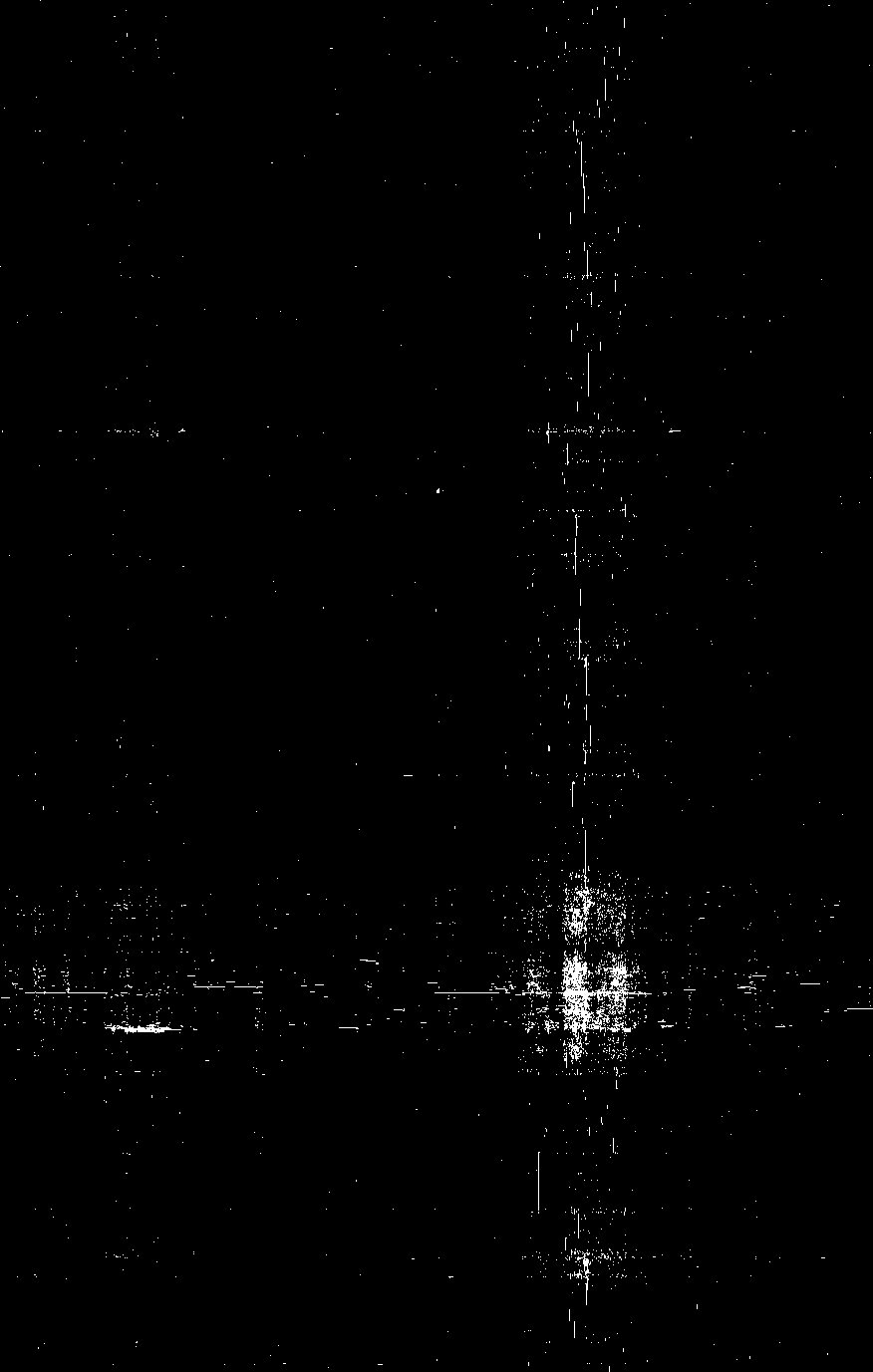满江红 念奴娇 水调歌头 吴潜 36 8 23 刘辰翁 4 16 26 辛弃疾 34 22 38 无名氏 42 42 40
 西江月 鹧鸪天 临江仙 刘辰翁 3 7 15 辛弃疾 17 63 24 无名氏 43 60 24 赵长卿 3 21 16
 浣溪沙 菩萨蛮 蝶恋花 张孝祥 30 22 5 石孝友 4 3 3 朱敦儒 8 5 黄庭坚 3 3 1 苏轼 46 22 15 陈亮 2 1 赵彦端 8 6 2 韩淲 36 16 5
 浣溪沙 菩萨蛮 蝶恋花 点绛唇 辛弃疾 20 22 12 2 无名氏 22 11 12 19 赵长卿 21 17 9 12 张元干 16 8 3 10
 清平乐 浣溪沙 菩萨蛮 蝶恋花 欧阳修 1 9 22 贺铸 7 27 12 9 毛滂 20 27 8 9 向子諲 7 25 4 2

par(mfrow = c(1, 2));
M = cor(mtcars);
order.AOE = corrMatOrder(M, order = "AOE");
M.AOE = M[order.AOE, order.AOE]
corrplot(M);
corrplot(M.AOE);
corrRect(c(4, 2, 5));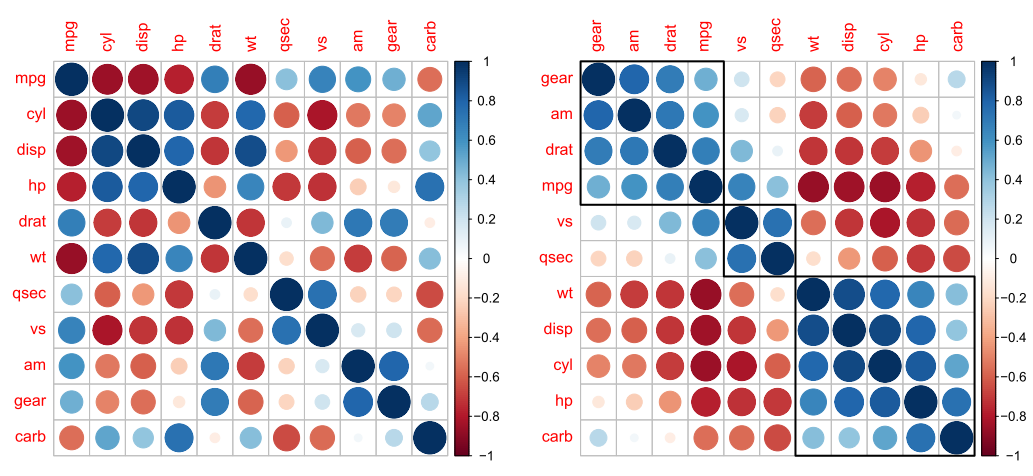======================开始跑题的分割线======================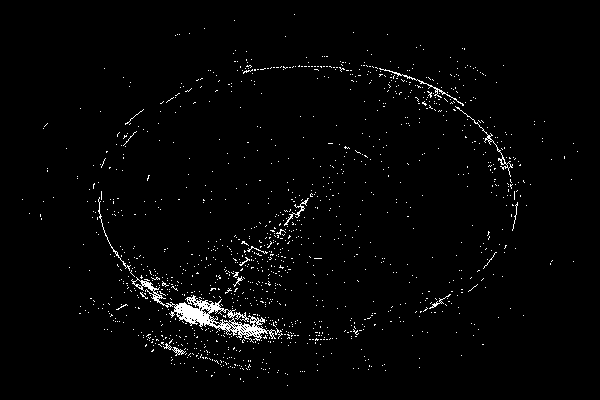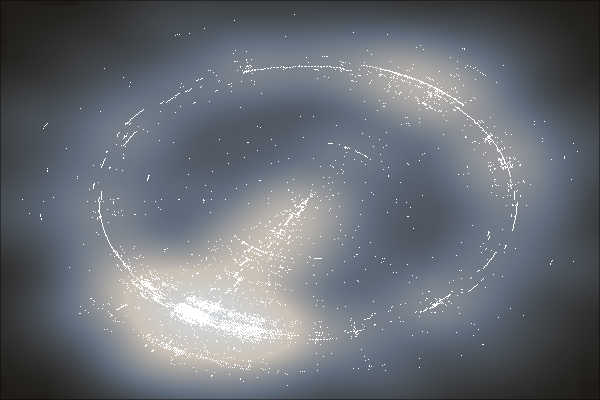visualizeMatrix = function(m)
{
m = m > 0;
par(mar = c(0, 0, 0, 0));
m = m[nrow(m):1, ];
image(1:ncol(m), 1:nrow(m), t(m), col = c("black", "white"),
useRaster = TRUE);
}
# 0-1矩阵
visualizeMatrix(tab[, ]);
# 矩阵排序
library(seriation);
set.seed(123);
ord = seriate(tab[, ] &gt; 0);
m = permute(tab[, ], ord);
visualizeMatrix(m);
# 极坐标计算
coord = which(m > 0, arr.ind = TRUE);
theta = (coord[, 2] - 1) / (max(coord[,2 ]) - 1) * 359 / 180 * pi;
rho = coord[, 1] / max(coord[, 1]);
x = rho * cos(theta);
y = rho * sin(theta);
# 极坐标图
par(bg = "black", mar = c(0, 0, 0, 0));
plot(x, y, col = "white", pch = ".");
# 平滑散点图
par(bg = "black", mar = c(0, 0, 0, 0));
mypalette = colorRampPalette(c("#1F1C17", "#637080",
"#CBC2B7", "#D2D6D9"),
space = "Lab");
smoothScatter(x, y, colramp = mypalette, nbin = 600, bandwidth = 0.1,
col = "white", nrpoints = Inf);